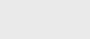3年前 (2020-10-06) |   抢沙发  384

- Niklaus Wirth，算法+数据结构=程序，1976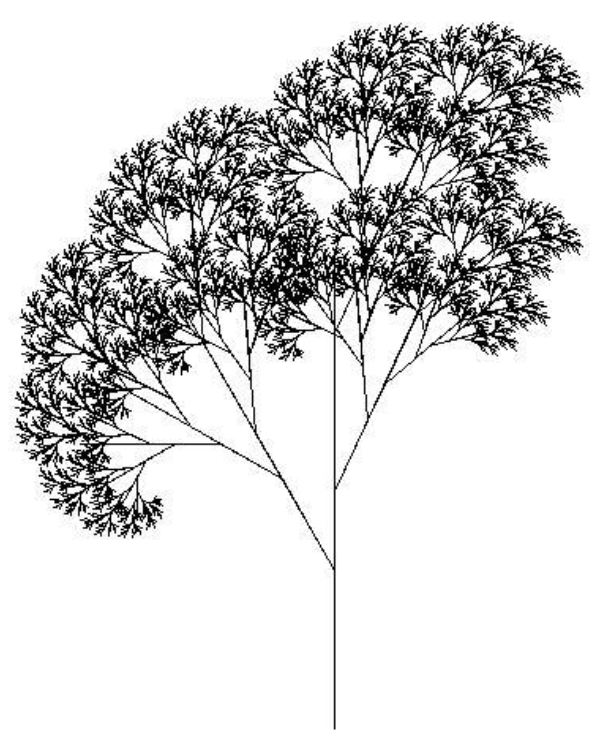## 递归数据类型

### 归纳定义数据

``data ListOfStrings = EmptyList | Cons String ListOfStrings``

``A natural number is either 1 or n+1, where n is a natural number.``

``````<expr> ::= <number>
| (<expr> * <expr>)
| (<expr> + <expr>)``````

### 共同定义的数据和核心词汇

``````A stream of strings is an object s such that:
tail(s) is a stream of strings.``````

`corecurson``coinduction`相关，可以用来计算（可能）无限对象的特定实例。作为一种编程技术，它最常用于惰性编程语言的上下文中，当程序输出的期望大小或精度未知时，它比递归更好。在这种情况下，程序既需要一个无限大（或无限精确）结果的定义，又需要一个获取结果有限部分的机制。计算前n个素数的问题是一个可以用`corecursive`程序（例如这里）解决的问题。

## 递归类型

### 结构递归与生成递归

-程序设计，Krishnat，2001

## 递归编程

### 递归过程

#### 阶乘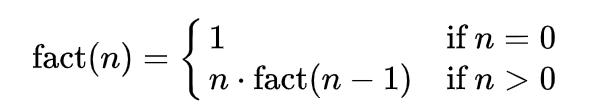``````function factorial is:

input: integer n such that n >= 0

output: [n × (n-1) × (n-2) × … × 1]

1. if n is 0, return 1
2. otherwise, return [ n × factorial(n-1) ]

end factorial``````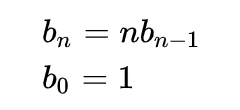``````b4           = 4 * b3

= 4 * (3 * b2)
= 4 * (3 * (2 * b1))
= 4 * (3 * (2 * (1 * b0)))
= 4 * (3 * (2 * (1 * 1)))
= 4 * (3 * (2 * 1))
= 4 * (3 * 2)
= 4 * 6
= 24``````

``````function factorial is:

input: integer n such that n >= 0

output: [n × (n-1) × (n-2) × … × 1]

1. create new variable called running_total with a value of 1

2. begin loop
1. if n is 0, exit loop
2. set running_total to (running_total × n)
3. decrement n
4. repeat loop

3. return running_total

end factorial``````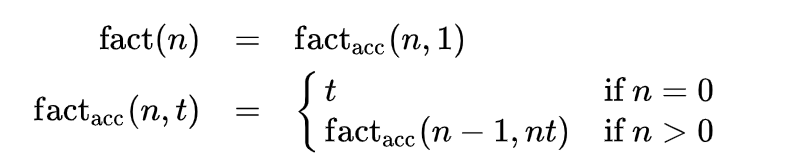#### 最大公约数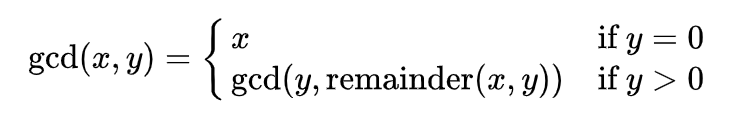``````function gcd is:
input: integer x, integer y such that x > 0 and y >= 0

1. if y is 0, return x
2. otherwise, return [ gcd( y, (remainder of x/y) ) ]

end gcd``````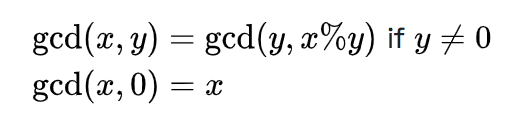``````Computing the recurrence relation for x = 27 and y = 9:
gcd(27, 9)   = gcd(9, 27% 9)
= gcd(9, 0)
= 9``````
``````Computing the recurrence relation for x = 111 and y = 259:
gcd(111, 259)   = gcd(259, 111% 259)
= gcd(259, 111)
= gcd(111, 259% 111)
= gcd(111, 37)
= gcd(37, 111% 37)
= gcd(37, 0)
= 37``````

``````Pseudocode (iterative):
function gcd is:

input: integer x, integer y such that x >= y and y >= 0

1. create new variable called remainder

2. begin loop
1. if y is zero, exit loop
2. set remainder to the remainder of x/y
3. set x to y
4. set y to remainder
5. repeat loop

3. return x

end gcd``````

#### 河內之塔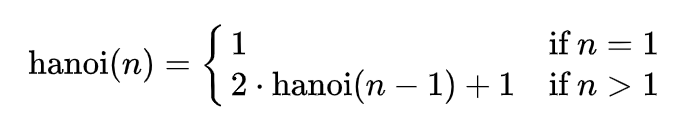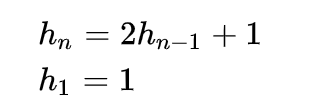``````Computing the recurrence relation for n = 4:
hanoi(4)     = 2*hanoi(3) + 1
= 2*(2*hanoi(2) + 1) + 1
= 2*(2*(2*hanoi(1) + 1) + 1) + 1
= 2*(2*(2*1 + 1) + 1) + 1
= 2*(2*(3) + 1) + 1
= 2*(7) + 1
= 15``````

``````function hanoi is:

input: integer n, such that n >= 1

1. if n is 1 then return 1

2. return [2 * [call hanoi(n-1)] + 1]

end hanoi``````

``````h1 = 1   = 21 - 1
h2 = 3   = 22 - 1
h3 = 7   = 23 - 1
h4 = 15  = 24 - 1
h5 = 31  = 25 - 1
h6 = 63  = 26 - 1
h7 = 127 = 27 - 1
In general:
hn = 2n - 1, for all n >= 1``````

#### 二进制搜索

``````/*
Call binary_search with proper initial conditions.

INPUT:
data is an array of integers SORTED in ASCENDING order,
toFind is the integer to search for,
count is the total number of elements in the array

OUTPUT:
result of binary_search

*/
int search(int *data, int toFind, int count)
{
//  Start = 0 (beginning index)
//  End = count - 1 (top index)
return binary_search(data, toFind, 0, count-1);
}

/*
Binary Search Algorithm.

INPUT:
data is a array of integers SORTED in ASCENDING order,
toFind is the integer to search for,
start is the minimum array index,
end is the maximum array index
OUTPUT:
position of the integer toFind within array data,
*/
int binary_search(int *data, int toFind, int start, int end)
{
//Get the midpoint.
int mid = start + (end - start)/2;   //Integer division

//Stop condition.
if (start > end)
return -1;
else if (data[mid] == toFind)        //Found?
return mid;
else if (data[mid] > toFind)         //Data is greater than toFind, search lower half
return binary_search(data, toFind, start, mid-1);
else                                 //Data is less than toFind, search upper half
return binary_search(data, toFind, mid+1, end);
}``````### 注册